## Saturday, 9 August 2014

### chapter 3 Special Triangle Theorems

Theorem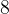$8$ Pythagoras’ Theorem
In a right – angled triangle the square of the hypotenuse is equal to the sum of the squares of the other two sides.Theorem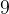$9$ (Sine Rule)
In a triangle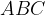$ABC$ where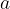$a$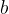$b$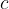$c$ are the lengths of the sides opposite the vertices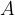$A$ ;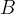$B$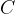$C$, respectively,$\dfrac{{\sin A}}{a} = \dfrac{{\sin B}}{b} = \dfrac{{\sin C}}{c}$Theorem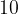$10$ (Cosine Rule)
In a triangle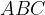$ABC$ where$a$$b$$c$ are the lengths of the sides opposite the vertices$A$$B$$C$ respectively,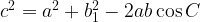${c^2} = {a^2} + b_1^2 - 2ab\cos C$Congruence of triangles
Triangles may be determined to be congruent by any of the following rules.
• SSS Rule If three sides of one triangle are equal to the three sides of another, then the triangles are congruent.
• SAS Rule If two sides and the included angle of one triangle are equal to the two sides and the included angle of another, then the triangles are congruent.
• ASA Rule If two angles and the included side of one triangle are equal to the two angles and the included side of another, then the triangles are congruent.
• RHS Rule If the hypotenuse and one other side of a right-angled triangle are equal to the hypotenuse and one side of another right-angled triangle, then the triangles are congruent.
Note that when we say two triangles$ABC$ and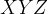$XYZ$ are congruent we mean that the correspondence of vertex$A$ to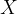$X$$B$ to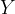$Y$ and$C$ to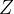$Z$ determines the congruence. We denote that two triangles$ABC$ and$XY Z$ are congruent by writing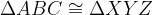$\Delta ABC \cong \Delta XYZ$.
Similarity of triangles
Each of the congruence rules has a corresponding similarity rule, by replacing side-length equality by proportionality. Thus, triangles may be determined to be similar by any of the following rules.
• SSS Rule If three sides of one triangle are in the same proportion as the three sides of another, then the triangles are similar.
• SAS Rule If two sides of one triangle are in the same proportion as the two sides of another, and the included angles of the sides that correspond are equal then the triangles are similar.
• AA Rule or AAA Rule If two angles of one triangle are equal to two angles of another, then the triangles are similar. (The equality of the two remaining corresponding angles are then necessarily equal.)
• RHS Rule If the hypotenuse and one other side of a right-angled triangle are in the same proportion as the hypotenuse and one side of another right-angled triangle, then the triangles are similar.
As with congruence, when we say two triangles$ABC$ and$XY Z$ are similar we mean that the correspondence of vertex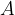$A$ to$X$$B$ to$Y$ and$C$ to$Z$determines the similarity. We denote that two triangles$ABC$ and$XY Z$ are similar by writing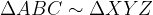$\Delta ABC \sim \Delta XYZ$.
Theorem$11$
If a line joins the midpoints of two sides of a triangle then that line is parallel to the third side and its length is equal to one half of the length of the third side.Theorem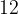$12$
A line parallel to one side of a triangle divides the other two sides in the same proportion.Theorem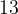$13$
The angle bisector of one side of a triangle divides the opposite side in the same ratio as the other two sides.